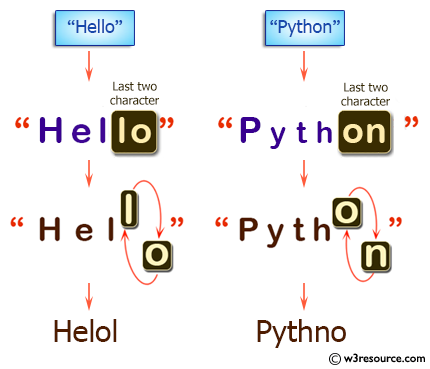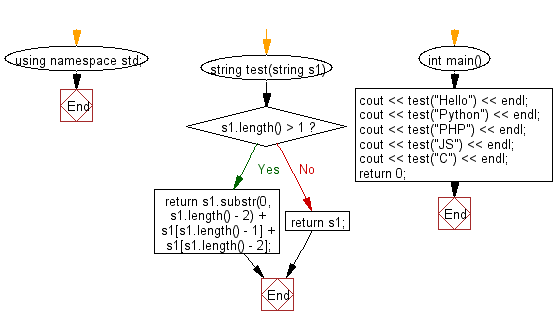﻿ C++ : New string swapping the last 2 characters of a string# C++ Exercises: Create a new string from a given string after swapping last two characters

## C++ Basic Algorithm: Exercise-73 with Solution

Write a C++ program to create a new string from a given string after swapping last two characters.

Sample Solution:

C++ Code :

``````#include <iostream>
using namespace std;

string test(string s1)
{
if (s1.length() > 1)
{
return s1.substr(0, s1.length() - 2) + s1[s1.length() - 1] + s1[s1.length() - 2];
}
else
{
return s1;
}
}

int main()
{
cout << test("Hello") << endl;
cout << test("Python") << endl;
cout << test("PHP") << endl;
cout << test("JS") << endl;
cout << test("C") << endl;
return 0;
}
``````

Sample Output:

```Helol
Pythno
PPH
SJ
C
```

Pictorial Presentation:Flowchart:C++ Code Editor:

Contribute your code and comments through Disqus.

What is the difficulty level of this exercise?

﻿